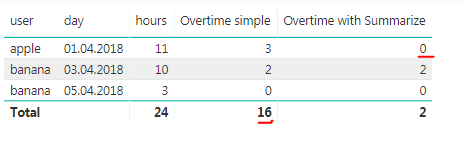cancel
Showing results for
Did you mean:Helper I

## Working with overtime calculation

Hi,

Tried searching the various other threads on this topic but they didn't really work for my case.

Users can have multiple entries per day depending on activity. I want to sum the total hours per day for each user, see if each user has gone over 8 hours and mark those overages as overtime.

Example of my raw data:

user day hours

 apple 01.04.2018 4 apple 01.04.2018 3 apple 01.04.2018 4 banana 03.04.2018 10 banana 05.04.2018 3

However, the problem is either the row value is wrong or the grand total is wrong. Here's what I have so far:```Overtime with Summarize =
SUMX(
SUMMARIZE(
Table1; Table1[hours]);
IF ( Table1[hours] > 8; Table1[hours] - 8; 0))```
```Overtime simple =
var overtime =
SUM(Table1[hours]) - 8

return
IF(overtime < 0; 0; overtime)```

Any help appreciated!

1 ACCEPTED SOLUTIONSuper User

OK, I think I got it:

```Measure 3 =
VAR overtime = SUM('#Overtime'[hours]) - 8
VAR tmpTable = SUMMARIZE('#Overtime',[user],[day],"Hours",SUM('#Overtime'[hours]))
VAR overtimeTotal = SUMX(tmpTable1,[Overtime])
RETURN IF(HASONEFILTER('#Overtime'[user]),IF(overtime<0,0,overtime),overtimeTotal)```

Become an expert!: Enterprise DNA
External Tools: MSHGQM
Latest book!:
Mastering Power BI 2nd EditionDAX is easy, CALCULATE makes DAX hard...
4 REPLIES 4Resolver IV

"IF ( Table1[hours] > 8; Table1[hours] - 8; 0)"  could be replaced by

MAX (Table1[hours] - 8; 0)Super User

OK, I think I got it:

```Measure 3 =
VAR overtime = SUM('#Overtime'[hours]) - 8
VAR tmpTable = SUMMARIZE('#Overtime',[user],[day],"Hours",SUM('#Overtime'[hours]))
VAR overtimeTotal = SUMX(tmpTable1,[Overtime])
RETURN IF(HASONEFILTER('#Overtime'[user]),IF(overtime<0,0,overtime),overtimeTotal)```

Become an expert!: Enterprise DNA
External Tools: MSHGQM
Latest book!:
Mastering Power BI 2nd EditionDAX is easy, CALCULATE makes DAX hard...Helper I

Thanks @Greg_Deckler! Works perfectly!

And thanks @MarkS on the tip.Super User

Become an expert!: Enterprise DNA
External Tools: MSHGQM
Latest book!:
Mastering Power BI 2nd EditionDAX is easy, CALCULATE makes DAX hard...